U.S. Department of Transportation
1200 New Jersey Avenue, SE
Washington, DC 20590
202-366-4000

Federal Highway Administration Research and Technology
Coordinating, Developing, and Delivering Highway Transportation Innovations

 REPORT This report is an archived publication and may contain dated technical, contact, and link information
 Publication Number:  FHWA-HRT-12-031    Date:  August 2012
 Publication Number: FHWA-HRT-12-031 Date: August 2012

# User’s Guide: Estimation of Key PCC, Base, Subbase, and Pavement Engineering Properties From Routine Tests and Physical Characteristics

PDF files can be viewed with the Acrobat® Reader®

## PCC Elastic Modulus Models

### Validation of Existing Models

Existing models correlate elastic modulus to compressive strength and unit weight. The following represent the regressed models using LTPP data for existing model forms: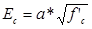Figure 55. Equation. Ec as a function of square root of compressive strength.

Regressed coefficients for figure 55 are as follows:

• a = 55,294.

Regression statistics for figure 55 are as follows:

• N = 514.
• R2 = 11.8 percent.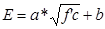Figure 56. Equation. Model form for E as a function of compressive strength with slope and intercept.

Regressed coefficients for figure 56 are as follows:

• a = 31,624.6.
• b = 2,013,192.

Regression statistics for figure 56 are as follows:

• N = 514.
• R2=11.8 percent.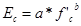Figure 57. Equation. Ec.

Regressed coefficients for figure 57 are as follows:

• a = 388,082.
• b = 0.2809.

Regression statistics for figure 57 are as follows:

• N = 514.
• R2 = 12.3 percent.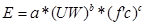Figure 58. Equation. E as function of unit weight and compressive strength.

Regressed coefficients for figure 58 are as follows:

• a = 80,849.3.
• b = 0.3648.
• c = 0.2527.

Regression statistics for figure 58 are as follows:

• N = 514.
• R2 = 10.8 percent.

The quality of prediction in the validated models is poor, as indicated by the R2 values reported for figure 55 through figure 58. This trend is common with elastic modulus models, especially considering that the data used in this study were not generated from controlled laboratory experiments. Also, while compressive strength is the most commonly used strength parameter and correlations with the compressive strength can be implemented most easily, there is an inherent drawback in correlating modulus to compressive strength. Modulus does not test the material to its limits. Instead, it is more indicative of the elastic deformational characteristics of the material. The data contain modulus measured at a wide range of ages. Therefore, the new models developed utilized other mix parameters that impact modulus, including age.

### Elastic Modulus Model 1: Model Based on Aggregate Type

The PCC elastic modulus model can be expressed as follows:

Figure 59. Equation. Prediction model 9 for Ec.

Where:

Ec = PCC elastic modulus, psi.
UW = Unit weight, lb/ft3.
f'c = Compressive strength.
Dagg = Regressed constant depending on aggregate type as follows:

• = 1.0 for andesite, limestone, and sandstone.
• = 0.9286 for basalt.
• = 1.0079 for chert.
• = 0.9215 for diabase.
• = 1.0254 for dolomite.
• = 0.8333 for granite.
• = 0.9511 for quartzite.

The development of the model required the use of a model form that accommodates aggregate type as categorical variables (assigned values of 1, 0). The values for Dagg were initialized to 1.0 at the start of the analyses and allowed to iteratively determine individual values for each aggregate type. The model had 71 observations, an R2 value of 35.8 percent, and an RMSE of approximately 500,000 psi.

The model indicates that the factor that accounts for the aggregate type, Dagg, has a value of 1.0 for andesite, limestone, and sandstone. Basalt, diabase, granite, and quartzite have lower Dagg values and therefore lower modulus values than mixes using andesite, limestone, and sandstone aggregates. Likewise, chert and dolomite have higher values. Table 18 provides details of the range of data used to develop the model.

Table 18. Range of data used for elastic modulus model based on aggregate type.

 Parameter Minimum Maximum Average Compressive strength 1,990 11,310 7,550 Unit weight 137 156 146 Elastic modulus 1,450,000 6,800,000 4,629,646

Figure 60 and figure 61 show the predicted versus measured plot and the residual plot, respectively. The R2 value is reasonable and therefore presented as a feasible model.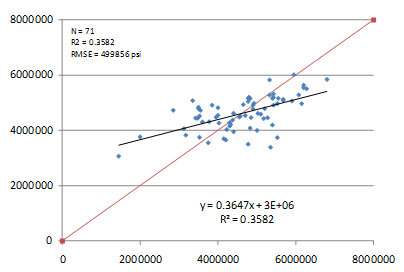Figure 60. Graph. Predicted versus measured for elastic modulus model based on aggregate type.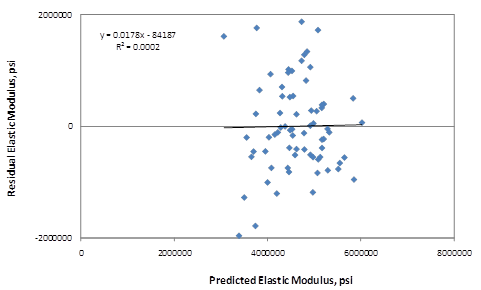Figure 61. Graph. Residual errors for elastic modulus model based on aggregate type.

### Elastic Modulus Model 2: Model Based on Age and Compressive Strength

The model can be expressed as follows:

Figure 62. Equation. Prediction model 10 for Ec,t.

Where:

Ec,t = Elastic modulus at age t,years.
f'ct = Compressive strength at age t, years.
t = Age at which modulus is determined, years.

The model uses 371 data points, has an R2 value of 26.14 percent, and an RMSE of about 900,000 psi. Table 19 shows the results of the nonlinear analysis, and table 20 provides details of the range of data used to develop the model.

Table 19. Regression statistics for elastic modulus model based on age and compressive strength.

 Parameter Constants Estimate Standard Error Approximate 95 Percent Confidence Limits a 59.0287 2.8881 53.3495 to 64.7079 b -0.2118 0.0284 -0.2677 to -0.1559

Table 20. Range of data used for elastic modulus model based on age and compressive strength.

 Parameter Minimum Maximum Average Compressive strength 1,990 12,360 7,361 Pavement age 0.0384 45.3836 14.0900 Elastic modulus 1,450,000 6,800,000 4,586,545

The measured versus predicted plot and the residuals plot for this model are shown in figure 63 and figure 64, respectively.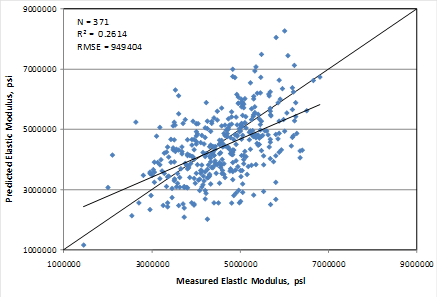Figure 63. Graph. Predicted versus measured for elastic modulus model based on age and compressive strength.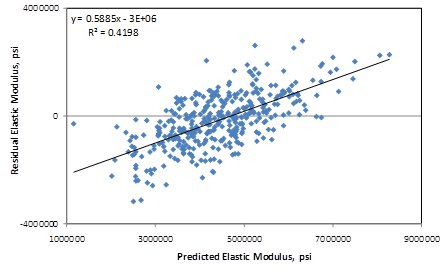Figure 64. Graph. Residual errors for elastic modulus model based on age and compressive strength.

### Elastic Modulus Model 3: Model Based on Age and 28-Day Compressive Strength

Since the 28-day compressive strength is usually available for PCC materials, a predictive model based on age and the 28-day compressive strength can be useful in many situations. The relationship developed for these variables can be expressed as follows:

Figure 65. Equation. Prediction model 11 for Ec,t.

Where:

Ec,t = Elastic modulus at age t, years.
f'c28-day = 28-day compressive strength.
t = Age at which modulus is determined, years.

The model used 46 data points, had an R2 value of 16.32 percent, and an RMSE of about 1,183,400 psi. Table 21 shows the results of the nonlinear analysis, and table 22 provides details of the range of data used to develop the model.

Table 21. Regression statistics for elastic modulus model based on age and 28-day compressive strength.

 Parameter Constants Estimate Standard Error Approximate 95 Percent Confidence Limits a 375.6 31.4592 312.5 439.3 b 0.00524 0.0714 -0.1388 -0.1492

Table 22. Range of data used for elastic modulus model based on age and 28-day compressive strength.

 Parameter Minimum Maximum Average 28-day compressive strength 3,034 7,912 5,022 Pavement age 0.0384 4.5288 0.9153 Elastic modulus 1,450,000 6,221,000 4,732,101

The measured versus predicted plot and the residuals plot for this model are shown in figure 66 and figure 67, respectively. This model uses data up to an age of 1 year. It is more appropriate for estimating the short-term modulus of a project and for supplementing strength estimates used to determine opening time for traffic.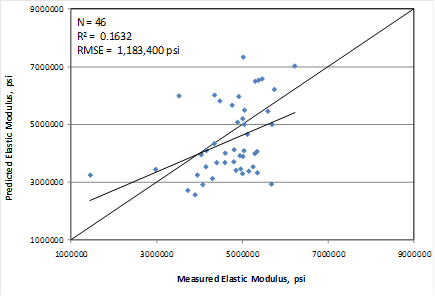Figure 66. Graph. Predicted versus measured for elastic modulus model based on age and 28-day compressive strength.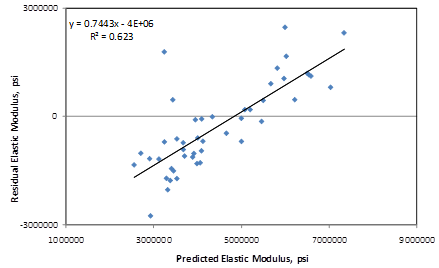Figure 67. Graph. Residual errors for elastic modulus model based on age and 28-day compressive strength.

### Limitations of Elastic Modulus Models

An examination of the statistics proposed for determining elastic modulus suggests that they do not possess the predictive ability of the other material parameters presented in this study. The models are considered fair but not excellent. They provide users with an option of moderate estimates when no information about the elastic modulus is available. Therefore, it is recommended that users exercise caution when using the predicted elastic modulus values for analyses.

Federal Highway Administration | 1200 New Jersey Avenue, SE | Washington, DC 20590 | 202-366-4000
Turner-Fairbank Highway Research Center | 6300 Georgetown Pike | McLean, VA | 22101